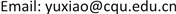PSASP，模型转换，自定义建模，电磁暂态仿真，PSCAD，自动布局, PSASP Model Conversion User-Defined Modeling Electromagnetic Transient Simulation PSCAD Auto Layout

1重庆大学，输配电装备及系统安全与新技术国家重点实验室，重庆

2中国电力科学研究院，北京1. 引言

2. 模型的对比

2.1. 同步发电机模型

2.2. 发电机控制系统模型

2.3. 负荷模型

PSASP中通常采用的是静态负荷和感应电动机负载，静态负荷的多项式如下：

{ P = P 0 [ A p ( V V 0 2 ) N p + B p ( V V 0 ) + C p ] ( ω ω 0 ) F p Q = Q 0 [ A q ( V V 0 2 ) N q + B q ( V V 0 ) + C q ] ( ω ω 0 ) F q (1)

{ P = P 0 ( V V 0 ) N p ( 1 + K p Δ f ) Q = Q 0 ( V V 0 ) N q ( 1 + K q Δ f ) (2)

2.4. 交流线模型

3. 基于自定义建模的模型转换方法3.1. 自定义建模

{ d x ' ( t ) d t = d x ' ' ( t ) d t = − 1 T x ' ( t ) + 1 T x ( t ) y ( t ) = x ' ' ( t ) + T 1 T 2 [ x ( t ) − x ' ( t ) ] (3)

{ x ' ( t ) = ( 1 − Δ t 2 T 2 ) x ' ( t − Δ t ) + K Δ t 2 T 2 [ x ( t − Δ t ) + x ( t ) ] 1 + Δ t 2 T 2     y ( t ) = x ' ' ( t ) + T 1 T 2 [ x ( t ) − x ' ( t ) ] (4)

3.2. 电磁暂态模型的自动生成

PSASP中的网络数据主要包括静态元件参数库和动态元件参数库，静态元件参数包括了元件所述数据组、编号、拓扑连接、支路参数信息。动态元件参数包括了发电机及其控制系统的型号、参数组等具体信息。自动生成电磁暂态模型的流程为：获取元件参数和拓扑信息，数据分组和元件模块化，自动布局和拓扑连接，生成PSC文件。

3.2.1. 数据的读取与转换

1) 参数的转换

2) 元件的模块化

3.2.2. 自动布局与拓扑连接

1) 力导向布局法

F r e p e l i n g = K q 1 q 2 R 2 (5)

F r e p e l i n g 是两个粒子所受的斥力， q 1 、 q 2 为两个粒子的质量； K 是斥力常量，以此调整全局的粒子之间的斥力，一般取值为−1。与此同时，某些粒子被一些能够产生类似弹簧胡克引力的“边”牵引着，“边”两端的粒子被紧紧牵连着。弹簧的引力计算如下式：

F e l a c t i c = H ( l c u r r e n t − l n a t u r e ) (6)

F e l a s t i c 是两个相连的粒子所受的弹簧力， H 是弹簧力的弹性系数， l c u r r e n t 是两个粒子当前的笛卡尔距离； l n a t u r e 是弹簧的自然长度，即两粒子的期望距离。在这些斥力与引力的不断作用下，初始时刻随机分布的粒子开始不断改变自己的位置，同时粒子所携带的能量也在不断消耗着，经过数次迭代计算过程，整个系统中的粒子的位置不再发生变化，系统中的能量达到最小，系统达到一种接近平衡的稳定状态。根据弹性势能，采用以下能量计算模型：

E = ∑ i = 1 n 0.5 H i ( l i − l i n a t u r e ) 2 (7)

2) 模块内部的布局

4. 实例验证

Active power of Yunyang substation outlet

Voltage of Yunyang substation outlet

5. 结语